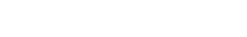# Binomial Theorem: Simple Definition, Formula, Step by Step Videos

## What is the Binomial Theorem?

The most common form of the binomial theorem (sometimes called a binomial expansion) used in statistics is simply a formula:The formula is used to figure out probabilities for binomial experiments (events that have two options, like heads or tails). You’ll find lots of binomial theorem help on this site, including how to solve the binomial formula (includes step by step video). Need help with a homework question? Check out our tutoring page!

## Binomials in Real Life

Binomial distributions are the results from experiments with two outcomes. The term “experiment” can mean a trial, a decision, or just a roll of the die. They are really just a measure of success (or failure). In other words, something happens, or it doesn’t. Will I live to 100, or won’t I? Will my car start, or won’t it? Can I pay my college tuition or not?
A simple example is buying a scratch-off lottery ticket.You’re either going to win, or you’re not. Knowing what your odds of winning are help you to decide if you’re going to buy a ticket.
Other examples:

• You find a parking space, or you don’t.
• You win Powerball, or you don’t.
• You get a birthday gift from your partner, or you don’t.
• A drug cures, or it doesn’t.
• A computer works, or it doesn’t.
• You get a paycheck, or you don’t.

You’d want to know if a computer is going to work before you buy it. Which means that you’ve probably used binomials without realizing it. Before you buy, you might have checked reviews. While a lot of review sites have ratings, they’re mostly in two categories: it’s great, or it sucks. Whether something works or not becomes more important in life or death situations; For example, taking a drug to see if it cures terminal disease. In fact, “life” and “death” are two outcomes, which makes anything that could result in death a binomial.

## Definition

Of course, you need a little math to describe it. A binomial distribution is the probability of something happening in an experiment. There are two variables, n and p.

• n: The number of times the experiment happens.
• p: The probability of one specific outcome.

For example, if you roll a die 20 times, then “n” is 20. If you buy ten lottery tickets, then n is 10. The odds of rolling a number (like 4) are 1 out of 6. So p=(1/6). And the odds of you winning a lottery are usually high, maybe one out of a million. In that case, p would equal (1/1 million).

## Example

If you were to roll a die 20 times, the probability of you rolling a six is 1/6. This ends in a binomial distribution of (n = 20, p = 1/6). For rolling an even number, it’s (n = 20, p = ½).

There are hundreds of ways you could measure success, but this is one of the simplest. Something works, or it doesn’t. It isn’t enough to say “maybe it will work”, especially if there’s money involved. For example, drugs cost billions to develop. Some medication cost patients over \$50,000 a year. Before the drug is made, the manufacturer wants to know if it will work. Before you a buy a drug, you’ll want to know if it’s going to work. They can all be measured with binomials.

Imagine a life without binomials. You wouldn’t know what the chances are of a drug curing you. You wouldn’t know what the odds were that you would get a side effect. You wouldn’t be able to compare different drugs. And you probably wouldn’t even be able to buy it in the first place. Why? Because buying something is a binomial experiment too. Can I afford it or can’t it? Do I have money in my wallet or don’t I? Do I have enough gas in my car? And so on…!

## Binomial Expansions and Series

The binomial formula in statistics is mostly used for counting and for calculating probabilities in experiments. A very similar technique, called binomial series expansion, is used in calculus for rewriting complicated functions into a simpler (binomial) form.

Check out my YouTube channel! I’ve got hundreds of videos up on all kinds of stats topics. If you’re taking a class, subscribe. Comments and suggestions are always welcome.

Have a question not covered here? Use the Site Search at the top of the page to find what you’re looking for.

## References

Agresti A. (1990) Categorical Data Analysis. John Wiley and Sons, New York.
Gonick, L. (1993). The Cartoon Guide to Statistics. HarperPerennial.
Kotz, S.; et al., eds. (2006), Encyclopedia of Statistical Sciences, Wiley.
Everitt, B. S.; Skrondal, A. (2010), The Cambridge Dictionary of Statistics, Cambridge University Press.
Vogt, W.P. (2005). Dictionary of Statistics & Methodology: A Nontechnical Guide for the Social Sciences. SAGE.
Lindstrom, D. (2010). Schaum’s Easy Outline of Statistics, Second Edition (Schaum’s Easy Outlines) 2nd Edition. McGraw-Hill Education

CITE THIS AS:
Stephanie Glen. "Binomial Theorem: Simple Definition, Formula, Step by Step Videos" From StatisticsHowTo.com: Elementary Statistics for the rest of us! https://www.statisticshowto.com/probability-and-statistics/binomial-theorem/
---------------------------------------------------------------------------Need help with a homework or test question? With Chegg Study, you can get step-by-step solutions to your questions from an expert in the field. Your first 30 minutes with a Chegg tutor is free!